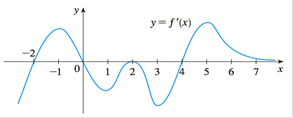Chapter 3.R, Problem 16E

Chapter
Section
Textbook Problem

# The figure shows the graph of the derivative f ′ of a function f.(a) On what intervals is f increasing or decreasing?(b) For what values of x does f have a local maximum or minimum?(c) Sketch the graph of f ″ .(d) Sketch a possible graph of f.To determine

(a)

To find:

The intervals where is f increasing or decreasing

Explanation

1) Concept:

Increasing / deceasing test:

I) If f(x) > 0, then the function is increasing

II) If f(x) <0,  then the function is decreasing.

If f(c) = 0, then the function has a critical point c, and hence, the function may not be increasing or decreasing at critical points.

2) Given:

The graph of a function y = f(x)

3) Calculation:

From the graph of a function y = fx, we see that

Function f is greater than 0 on (-2, 0) and 4, .

Therefore, by the increasing or decreasing test, the function f is increasing on (-2, 0) and (4, )

To determine

(b)

To find:

Values of x for which f has local maximum or minimum

To determine

(c)

To sketch:

The graph of f

To determine

(d)

To sketch:

A possible graph of f

### Still sussing out bartleby?

Check out a sample textbook solution.

See a sample solution

#### The Solution to Your Study Problems

Bartleby provides explanations to thousands of textbook problems written by our experts, many with advanced degrees!

Get Started

#### solve the equation by using the quadratic formula. 135. 4x2 + 5x 6 = 0

Applied Calculus for the Managerial, Life, and Social Sciences: A Brief Approach

#### Can the matrices in Problems 5-8 be transition matrices? If not, why? 6.

Mathematical Applications for the Management, Life, and Social Sciences

#### Evaluate the limit, if it exists. limx4x2+95x+4

Single Variable Calculus: Early Transcendentals

#### j × (−k) = i −i j + k −j − k

Study Guide for Stewart's Multivariable Calculus, 8th

#### In Exercises 3538, find the transpose of each matrix. 

Finite Mathematics for the Managerial, Life, and Social Sciences